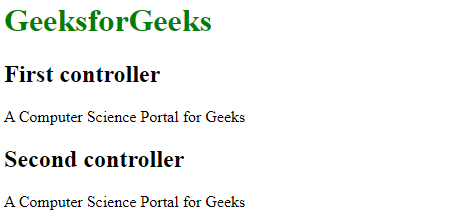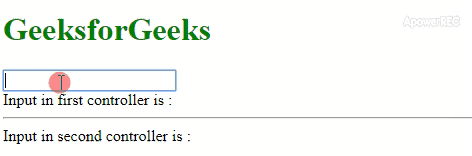# How to share data between controllers in AngularJS ?

• Last Updated : 30 Jan, 2020

The task is to share data variable between two or more controllers by using AngularJS. There are many procedures to achieve this. Here we will discuss the most popular ones.

Approach: To share data between the controllers in AngularJS we have two main cases:

• Share data between parent and child: Here, the sharing of data can be done simply by using controller inheritance as the scope of a child controller inherits from the scope of the parent controller.
• Share data between controllers without having relation: Here, the sharing of data can be done through a few ways some of them are:

By using rootScope variable: We can use the rootScope variable to hold shared data and then can reference it from any controller. Here, at the starting of the Angular app, we initialized the rootScope variable with some value and then refer it from every controller and thus bind scope variables in both controllers to the rootScope variable.

• Example:

 ```<``html``>`` ` `<``head``>``    ``<``title``>``        ``Angular JS sharing data using rootScope``    ````    ``<``script` `src``=``"https://ajax.googleapis.com/ajax/libs/angularjs/1.3.14/angular.min.js"``>``    `````` ` `<``body``>``    ``<``h1` `style``=``"color:green;"``> ``        ``GeeksforGeeks ``    ```` ` `    ``<``div` `ng-app``=``"mainApp"``>``        ``<``div` `ng-controller``=``"firstcontroller"``>``            ``<``h2``>First controller``            ``<``p``>{{firstvalue}}``        ````        ``<``div` `ng-controller``=``"secondcontroller"``>``            ``<``h2``>Second controller``            ``<``p``>{{secondvalue}}``        ````    ```` ` `    ``<``script``>``        ``var mainApp = angular.module("mainApp", []);`` ` `        ``mainApp.run(function(\$rootScope) {``            ``\$rootScope.value = ``               ``'A Computer Science Portal for Geeks';``        ``});``        ``mainApp.controller('firstcontroller',``            ``function(\$scope, \$rootScope) {``                ``\$scope.firstvalue = \$rootScope.value;``            ``});``        ``mainApp.controller('secondcontroller',``            ``function(\$scope, \$rootScope) {``                ``\$scope.secondvalue = \$rootScope.value;``            ``});``    `````` ` `                    `

• Output:By using factory or service: The \$rootscope method is not preferred for data transfer or sharing data because it has a global scope that is available for the entire application. So, we use another method in which we create a factory or service to hold share data. AngularJS factories and services are JS functions that perform a specific task containing both methods & properties and can be injected in other components (e.g. your controllers) using dependency injection. In this way we can define a shared variable in a factory, inject it in both controllers and thus bind scope variables in both controllers to this factory data.

• Example:

 ```<``html``>`` ` `<``head``>``    ``<``title``>``        ``Angular JS sharing data using factory``    ````    ``<``script` `src``=``"https://ajax.googleapis.com/ajax/libs/angularjs/1.3.14/angular.min.js"``>``    `````` ` `<``body``>``    ``<``h1` `style``=``"color:green;"``> ``        ``GeeksforGeeks ``    ```` ` `    ``<``div` `ng-app``=``"mainApp"``>``        ``<``div` `ng-controller``=``"FirstController"``>``            ``<``input` `type``=``"text"` `ng-model``=``"value.name"``>``            ``<``br``> Input in first controller is : {{value.name}}``        ````        ``<``hr``>``        ``<``div` `ng-controller``=``"SecondController"``>``            ``Input in second controller is : {{value.name}}``        ````    ```` ` `    ``<``script``>``        ``var mainApp = angular.module("mainApp", []);`` ` `        ``mainApp.factory('Fact', function() {``            ``return {``                ``name: ''``            ``};``        ``});`` ` `        ``mainApp.controller('FirstController',``                        ``function(\$scope, Fact) {``            ``\$scope.value = Fact;``        ``});`` ` `        ``mainApp.controller('SecondController',``                        ``function(\$scope, Fact) {``            ``\$scope.value = Fact;``        ``});``    `````` ` `                    `

• Output:My Personal Notes arrow_drop_up